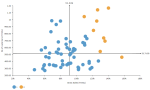# DCPY.AGGLOCLUST(n_clusters, affinity, linkage, columns) Follow

Agglomerative clustering is a hierarchical clustering algorithm that builds nested clusters by merging or splitting them successively. This implementation uses bottom-up approach, where each observation starts in its own cluster, and then clusters are successively merged together based on the linkage metric.

###### Parameters
• n_clusters – Number of clusters that the algorithm should find, integer (default 2).

• affinity – Metric used to compute the linkage (default euclidean)
• Possible values: euclidean, l1, l2, manhattan, cosine, precomputed.

• If a linkage parameter is set to ward, only euclidean is supported.

• linkage – Determines which distance to use between sets of data points, which is used for merging clusters. Possible values are ward, complete, and average (default ward).

• ward – Minimizes variance of the clusters being merged.

• average – Uses an average distance between two sets.

• complete – Uses maximum distances.

Example: DCPY.AGGLOCLUST(2, ’euclidean’, ’ward’, sum([Gross Sales]), sum([No of customers])) used as a calculation for the Color field of the Scatterplot visualization.###### Input data
• Numeric variables are automatically scaled to zero mean and unit variance.
• Character variables are transformed to numeric values using one-hot encoding.
• Dates are treated as character variables, so they are also one-hot encoded.
• Size of input data is not limited, but many categories in character or date variables increase rapidly the dimensionality.
• Rows that contain missing values in any of their columns are dropped.
###### Result
• Column of integer values starting with 0, where each number corresponds to cluster assigned to each record (row) by the agglomerative clustering algorithm.
• Rows that were dropped from input data (due to containing missing values) are not assigned to a cluster.
###### Key usage points
• Similar to K-means, this method is a good choice when dealing with spherical clusters and normal distributions.
• Can yield better clustering results than K-means.
• Use it when you know that approximate number of clusters should be higher and when the number of data points do not exceed few thousands.
• When the clusters are ellipsoidal rather than spherical (variables are correlated within a cluster), the clustering result may be misleading.
• High in time complexity, not scalable with higher number of records.
• Must know the number of clusters.
###### Example

For the whole list of algorithms, see Data science built-in algorithms.